# mandelbrot diagrams

this web page is based on an article by cliff ramshaw

Counting becomes increasingly abstract the more you go into it. Very large numbers, for example, have less real meaning than small ones. It becomes increasingly difficult to find analogies for decimals and minus numbers and comparisons with reality become very flimsy indeed when doing certain operations on negative numbers. Any pretence at reality finally vanishes when you find the square root of a negative number and it is then called an imaginary number or 'complex' number. The unit complex number is called 'i', which is the square root of minus one.
Complex numbers can be represented on an 'Argand diagram', a graph with the real part of a number along the x axis and the imaginary part on the y axis. This is also known as 'the complex plane'. Argand diagrams are normally used to describe vectors, but they can also be used to make mandelbrot diagrams.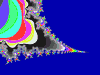The Mandelbrot test is performed as follows: take the complex number corresponding to each point on the complex plane in turn. Square this number , then add the original number. Square the result, and again add the original number. Do this forever, or until the result exceeds two .
To square a number which is in the form (a + b) you use the mathematical formula: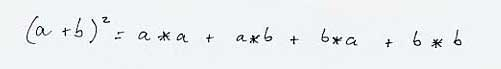So, taking this into account, imagine the complex number 3 + 2i.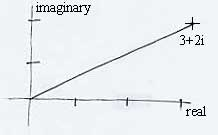Squaring this gives: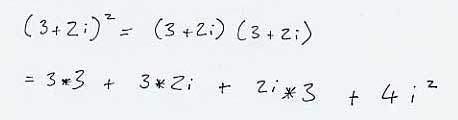Because i squared is -1 so.....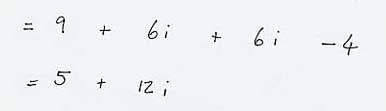Adding the number we first thought of, (3+2i), gives 8+14i.
Now test if the magnitude of this complex number is greater than two. The magnitude is found by using Pythagoras' Theorem: squaring and adding the real and imaginary parts, then square rooting the whole lot. Note that we don't go squaring the 'i' again, just the number in front of it. So the magnitude of the number above is the square root of (8 * 8)+(14 * 14) which is roughly 16, substantially larger than 2. Hence (2 + 3i) is not in the Mandelbrot set.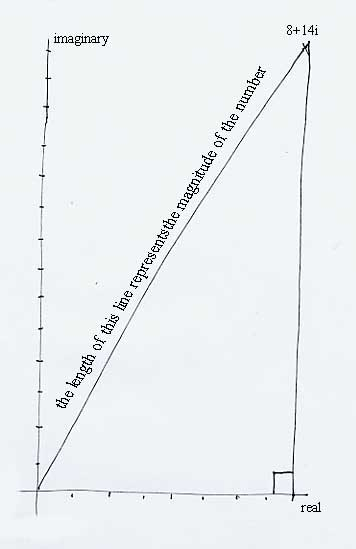For other numbers it might not be so simple to decide. To be completely sure that a number is in the set, we have to repeat the squaring and adding procedure for ever, just to make sure that the result never goes above 2. In practice, however, we content ourselves with an approximate solution. We repeat the process for a certain number of times (for example 16 times). If the number still remains below 2, then we give up, assume that it's in the Mandelbrot set and mark it with a black dot on the screen. Otherwise, the dot is coloured according to how many iterations it took before the result went above two.
You will get the classic Mandelbrot image by checking between -1.5 and +1.5 in both the real and imaginary axes.
A good language for writing the program is QBASIC because it has very well-written help. It used to be routinely included in Windows and it will run in DOS on a pc in which Windows is no longer working. It is very compact (250k) so can be put on a floppy disc along with the help file, and run from the A drive if necessary. There is no setup procedure: you just run it.
It is a good idea to start by writing simpler prototype programs: for example a program which draws a (small) square of a chosen colour. QBASIC has 16 colours numbered from 0 to 15 which is a about right for the maximum number of iterations before giving up.
This is a prototype QBASIC program

SCREEN 9
LINE(100,200)-(105,205),3,BF

It draws a small turquoise (3 means 'turquoise') square, the top-left coordinates being 100,200; the bottom-right being 105,205. BF means 'Background Fill' (filled shape rather than just outline).
Having done this write one which moves the origin to the center of the screen and draws a pattern (any pattern) of squares in the range -1.5 to +1.5 in both the x and y axes.
Experiment with different rules for deciding the colour of the squares. Start with simple ones like 'is the x value more than one' etc then try more complicated ones like 'how many times do I have to square the x value before it's more than 2'. By the time you've done some of these you'll be in a position to make your Mandelbrot program work.
Example of pseudocode
'Fractint' is a very good fractal-drawing program, it is freely available on the internet, it too will run with just DOS. Old versions were very compact and could be run off the A drive. It gives a very good overview of the subject.
fractal animation
john & betty's journey into complex numbers
wikipedia on fractals
online fractal generator
my own footling attempt: works by moving mouse & clicking. 'Refresh' to go again. It took me about two weeks to write.
movie depicting infinite detail.

whacky drawing